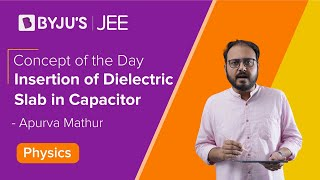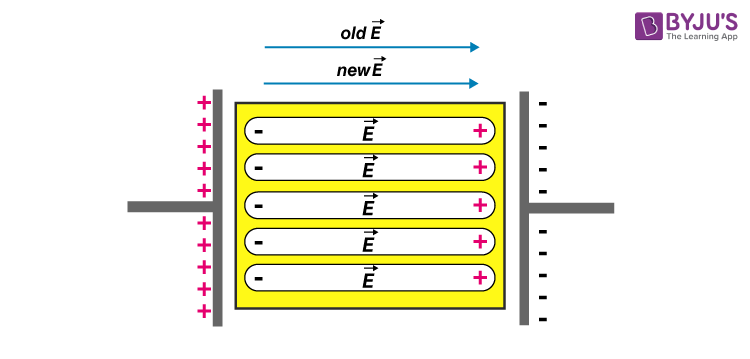# Know the Effect of Dielectric on Capacitance

We have read about the working of a parallel plate capacitor. We know that capacitance’s value depends on the material or the medium between the two plates. In this section, we will learn in detail how the capacitance of a parallel plate capacitor changes when a dielectric medium is inserted between its plates. In this section, let us learn more about the effect of dielectric on capacitance in an interesting way.

## Effect of Dielectric on Capacitance

To know the effect of dielectric on capacitance let us consider a simple capacitor with parallel plates of area A, separated by a distance d, we can see that the charge on each plate is +Q and –Q for a capacitor with charge Q. As the area of the plate is A, the corresponding charge density can be given as ±σ. Where,

$$\begin{array}{l}\sigma =\frac{Q}{A}\end{array}$$
When the two plates have a vacuum between them, potential difference across the capacitor can be given as,
$$\begin{array}{l}V_{0}=E_{0}d=\frac{\sigma }{\varepsilon _{0}}d\end{array}$$
The capacitance of the capacitor can thus be given as,
$$\begin{array}{l}C_{0}=\frac{Q}{V_{0}}=\varepsilon _{0}\frac{A}{d}\end{array}$$

## Understand how the dielectric slab of a capacitor affects the voltage and electric field of a capacitor by watching the videoLet us consider another capacitor with the exact specifications as taken before. Let us insert a dielectric between the plates such that it fully occupies the space between the plates. As the dielectric enters the field between the plates, it gets polarized by the field, and the charges get arranged such that they act as two charged sheets with a surface charge density of σp and – σp, as shown in the figure below.The net surface charge density then becomes equivalent to ±(σ – σp).

The potential difference across the capacitor can thus be given as,

$$\begin{array}{l}V=Ed=\frac{\sigma -\sigma _{p}}{\varepsilon _{0}}d\end{array}$$

In the case of linear dielectrics, we can say that σp is proportional to E0 and hence it is proportional to σ. Thus, we can say that the value (σ – σp) is also proportional to σ. Mathematically,

$$\begin{array}{l}\sigma -\sigma _{p}=\frac{\sigma }{K}\end{array}$$

Where K is a constant whose value depends upon the dielectric medium selected. The potential energy across the capacitor can this be written as,

$$\begin{array}{l}V=\frac{\sigma d}{\varepsilon _{0}K}=\frac{Qd}{A\varepsilon _{0}K}\end{array}$$

And the capacitance between the plates can be given as,

$$\begin{array}{l}C=\frac{Q}{V}=\frac{\varepsilon _{0}KA}{d}\end{array}$$

Here ε0K is the permittivity of the medium, which can also be given as,

$$\begin{array}{l}\varepsilon =\varepsilon _{0}K\end{array}$$

Here the value K is the permittivity of the medium such that, for a given medium,

$$\begin{array}{l}K=\frac{\varepsilon }{\varepsilon _{0}}\end{array}$$

And the ratio of the capacitance of the capacitor with a dielectric medium to the capacitor with a vacuum between the plates can be given as,

$$\begin{array}{l}K=\frac{C}{C _{0}}\end{array}$$

#### Important JEE questions on Electrostatic Potential and Capacitance#### Know About Dielectric in a Capacitors

## Frequently Asked Questions – FAQs

Q1

### Can the capacitor store the charge?

Yes, the capacitor can store the charge.

Q2

TRUE.

Q3

### Give some examples of dielectric materials.

Examples of dielectric materials are ceramic, mica, plastics, and glass.
Q4

### The ratio of the capacitance of the capacitor with a dielectric medium to the capacitor with a vacuum between the plates is given as?

$$\begin{array}{l}K=\frac{C}{C _{0}}\end{array}$$
Q5

### When the two plates have a vacuum between them, potential difference across the capacitor is given as?

$$\begin{array}{l}C_{0}=\frac{Q}{V_{0}}=\varepsilon _{0}\frac{A}{d}\end{array}$$

Hope you have understood the effect of dielectric on capacitance. Stay tuned with BYJU’S to learn more about the effect of dielectric on a capacitor and other related topics.

Test Your Knowledge On Effect of Dielectric on Capacitance!